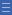## Featured resource

Home > Topdrawer > Fractions > Good teaching > Equivalence

# Equivalence

Equivalence

Understanding the equivalence of fractions is fundamental to the development of proportional reasoning. It begins with exploring physical models of fractions, gradually connecting with knowledge of factors and multiples.

The concept of equivalence begins with noticing the equality of representations of particular fractions. Often an area model is used, like partitioning circles into equal parts.

An essential skill at this stage is that of re-unitising: subdividing a whole into smaller and smaller parts. Focussing on fractions with related denominators at first — halves, quarters and eighths — makes these relationships easier to notice and explain.

Activities with linear models, like folding paper strips or placing familiar fractions on a number line, will help develop flexibility in thinking about what equivalence means.

Modelling fractions using grids and arrays supports the exploration of fractions with related denominators, and makes the connection to factors and multiples knowledge. This lays the foundation for effective strategies for creating equivalent fractions without relying on physical models.

Further information about students’ thinking about equivalence can be found in the article Assessing Students' Understanding of Fraction Equivalence on the AAMT website.

Yes

Yes

Name Class SectionLinear models Folder 17Grids and arrays Folder 17Year 4: Investigate equivalent fractions used in contexts Infobox 3Year 6: Compare fractions with related denominators and locate and represent them on a number line Infobox 3Source Infobox 3